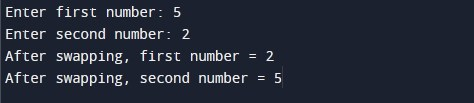# C Program to Swap two numbers Using Function

In this tutorial you will learn about the C Program to Swap two numbers Using Function and its application with practical example.

In this tutorial, we will learn to create a program to swap two numbers using function (call by reference) in C programming language.

Prerequisites

Before starting with this tutorial we assume that you are best aware of the following C programming topics:

• C Data Types
• C Variables
• C Input Output
• C Operators
• C Function
• C Pointer

## Program to Swap Numbers Using Function ( call by reference )

In this program we will swap two integer numbers using function (call by reference). We would first declare and initialize the required variables. Next, we would prompt user to input two integer numbers. Later in the program we will swap numbers by calling a function( call by reference ). Finally, we will display number values after swapping of numbers.

Output:-In the above program, we have first defined a function called swap() as following:

swap(int *a, int *b) :- Function accept two parameter as call by reference and swap them.

In the above program, we have first declared and initialized a set variables required in the program.

• num1 = it holds the first integer number value
• num2 = it holds the second integer number value

In the next statement user will be prompted to enter the two integer number values which will be assigned to variable ‘num1’ and ‘num2’ respectively. Next, we will swap two integer numbers using swap() function passing ‘num1’ and ‘num2’ by reference.

In this tutorial we have learn about the C Program to Swap two numbers Using Function and its application with practical example. I hope you will like this tutorial.